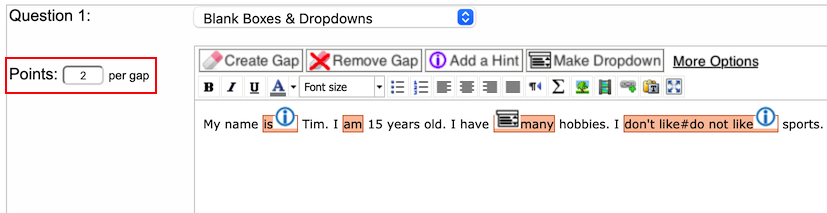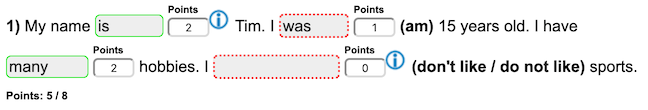# Change the number of points for gaps

You can now change the number of points that are given for gaps per question. By default, it’s 1 point for each gap that was answered correctly. But if for instance, the gaps in one question are more difficult than for other questions, you might want to give 2 points per gap:If you think not all gaps are worth 2 points in the above question, you would have to split the text into several questions. You can also change the number of points per gap manually when grading:In the above example, we have 4 gaps, each worth 2 points, so the total number of possible points for this question is 8. The student answered 2 gaps correctly and I manually gave him 1 point after he submitted the answers for the second gap as at least he got the verb correct (although in the wrong tense). So after clicking the button “Submit Points and Corrections” which you will see at the bottom of the page (see Statistics & Grades), it will update the number of points for that question to 5.